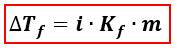# Problem: What is Kf for water if the freezing point was lowered to -12°C once 30 g of Sodium Chloride was added to 150 g of water?

###### FREE Expert Solution
89% (183 ratings)
###### FREE Expert Solution

We’re being asked to find the Kf (freezing point depression constant) of water if its freezing point decreased to -12°C when NaCl was dissolved in it. We’re going to use the Freezing Point Depression equation to calculate for Kf.∆Tf = change in freezing point = Tf pure solvent –Tf solution
Kf = freezing point depression constant
i = van' t Hoff factor of the solute = no. of ions
m = molality

Let’s first calculate the molality of the solution.

89% (183 ratings)###### Problem Details

What is Kf for water if the freezing point was lowered to -12°C once 30 g of Sodium Chloride was added to 150 g of water?

What scientific concept do you need to know in order to solve this problem?

Our tutors have indicated that to solve this problem you will need to apply the Freezing Point Depression concept. If you need more Freezing Point Depression practice, you can also practice Freezing Point Depression practice problems.

What is the difficulty of this problem?

Our tutors rated the difficulty ofWhat is Kf for water if the freezing point was lowered to -1...as high difficulty.

How long does this problem take to solve?

Our expert Chemistry tutor, Dasha took 6 minutes and 51 seconds to solve this problem. You can follow their steps in the video explanation above.

What professor is this problem relevant for?

Based on our data, we think this problem is relevant for Professor Chakraborty's class at FIU.